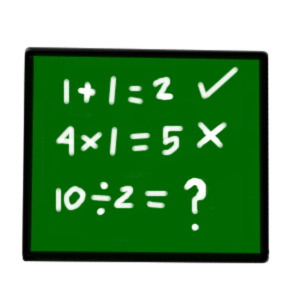# Numbers and Symbols for Maths

The short form of mathematics in British English is maths and in American English it's math.Can you add, subtract and do your multiplication tables in English? Before you can do the math you need to understand the question. Learning English for math means learning numbers, words, and symbols.

1 + 2 = 3
Five plus three equals eight.
One and six is seven.

## Subtraction -

7 - 6 = 1
Three minus two equals one.
Eighteen subtract four is fourteen.

## Multiplication x

5 x 6 = 30
Seven times three equals twenty-one.
Two multiplied by three is six.

## Division ÷

9 ÷ 3 = 3
Six divided by three equals two.
Five goes into fifteen three times.

## Other Maths Symbols:

 < less than > greater than ¼ fractions % percent 90° degrees 1.666 decimals

## Word problems

Word problems are maths questions written in sentences. Here are some for you to try!

1. Two monkeys collected 15 bananas. One monkey collected 7 bananas. How many bananas did the other monkey collect?
2. Johnny had six apples on Sunday. On Monday, Johnny gave Sally half of his apples. On Tuesday, he ate one of his apples. How many apples did Johnny have left on Wednesday?
3. Three women thought they were pregnant with twins. Two of the women were right. The third woman was surprised with triplets. How many babies were born?

Answers: Question 3: 7. Question 1: 8. Question 2: 2.